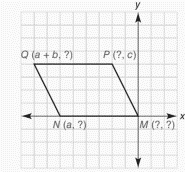Chapter 10.CR, Problem 30CR### Elementary Geometry for College St...

6th Edition
Daniel C. Alexander + 1 other
ISBN: 9781285195698

#### Solutions

Chapter
Section### Elementary Geometry for College St...

6th Edition
Daniel C. Alexander + 1 other
ISBN: 9781285195698
Textbook Problem
1 views

# In Review Exercise 27 to 30, supply the missing coordinates for the vertices, using as few variables as possible.Parallelogram M P Q N

To determine

To find:

The missing coordinates for the vertices.

Explanation

The given figure is shown below.

From the figure, the following observations are made.

Since, the vertex M is at the origin.

The coordinates of M0, 0.

Now, consider the vertex N.

Here, x-coordinate is given as a.

In N vertex, observe where it lies on y- axis.

Since, it is at 0, the vertex N has the coordinates a, 0.

Consider the vertex Q.

In the y-axis, it lies on the same line where P lies.

y-coordinate is same as y-coordinate of P.

y-coordinate is c

### Still sussing out bartleby?

Check out a sample textbook solution.

See a sample solution

#### The Solution to Your Study Problems

Bartleby provides explanations to thousands of textbook problems written by our experts, many with advanced degrees!

Get Started

#### 13. Write the equation of the line tangent to

Mathematical Applications for the Management, Life, and Social Sciences

#### True or False: is a convergent series.

Study Guide for Stewart's Multivariable Calculus, 8th

#### Find each value of x. 2log25=x

College Algebra (MindTap Course List)